# Class 11 Maths NCERT Solutions for Chapter 8 Binomial Theorem Exercise 8.2### Binomial Theorem Exercise 8.2 Solutions

1. Find the coefficient of x5 in (x + 3)8

Solution

It is known that (r + 1)th term, (Tr+1), in the binomial expansion of (a + b)n is given by Tr+1 = nCr an-rbr.
Assuming that x5 occurs in the (r + 1)th term of the expansion (x + 3)8 , we obtain
Tr+1 = 8Cr x8-r 3r.
Comparing the indices of x in x5 and in Tr+1, we obtain
r = 3
Thus, the coefficient of x5 is 8Cr (3)3 = (8!/3!5!) × 33 = (8.7.6.5!/3.2.5!).33 = 1512

2. Find the coefficient of a5 b7 in (a - 2b)12 .

Solution

It is known that (+ 1)th term, (Tr+1), in the binomial expansion of (b)n is given by Tr+1 = nCr an-r br .
Assuming that a5 b7 occurs in the (r + 1)th term of the expansion (a - 2b)12 , we obtain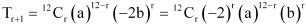Comparing the indices of a and b in a5 b7 and in Tr+1 , we obtain
r = 7
Thus, the coefficient of a5 b7 is 12C7 (-2)7 = (-12!/7!5!) .27 = (12.11.10.9.8.7!/5.4.3.2.7!) 27 = -(792)(128) = -101376

3. Write the general term in the expansion of (x2 - y)6

Solution

It is known that the general term Tr+1{which is the (r+1)th term} in the binomial expansion of (a + b)n is given by Tr+1 = nCr an-r br .
Thus, the general term in the expansion of (x2 - y6) is
Tr+1 = 6Cr (x2 )6-r (-y)r . (-1)r  6Cr x12-2r yr

4. Write the general term in the expansion of (x2 – yx)12x ≠ 0

Solution

It is known that the general term Tr+1 {which is the (r + 1)th term} in the binomial expansion of (a + b)2 is given by Tr+1 = nCr an-r br .
Thus, the general term in the expansion of (x2 - yx)12 is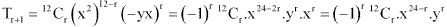5. Find the 4th term in the expansion of (x – 2y)12 .

Solution

It is known that (r + 1)th term, Tr+1, in the binomial expansion of (a + b)n is given by Tr+1 =  nCr an-r br .
Thus, the 4th term in the expansion of (x - 2y)12 is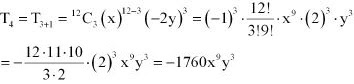6. Find the 13th term in the expansion of (9x - 1/3√x)18 , x ≠ 0.

Solution

It is known that (r + 1)th term, (Tr+1 ), in the binomial expansion of (a + b)n is given by Tr+1 = nCr an-r br.
Thus, 13th term in the expansion of (9x - 1/3√x)18 is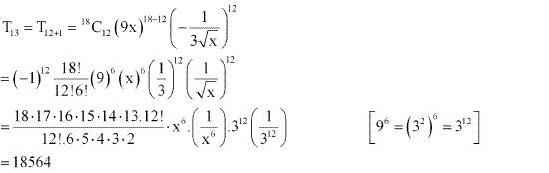7. Find the middle terms in the expansions of (3 - x3 /6)7 .

Solution

It is known that in the expansion of (a + b)n, if n is odd, then there are two middle terms, namely, [(n+1)/2]8 term and [(n+1)/2 + 1)th term.
Therefore, the middle terms in the expansion of [3 - x3 /6]7 are [(7+1)/2]th = 4th term and [(7+1)/2 + 1)th = 5th term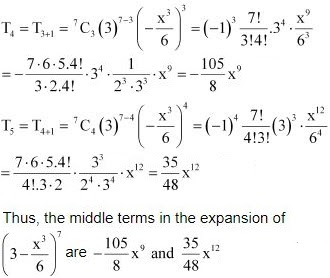8. Find the middle terms in the expansions of (x/3 + 9y)10

Solution

It is known that in the expansion (a + b)n , if n is even , then the middle term is (n/2 + 1)th term.
Therefore, the middle term in the expansion of (x/3 + 9y)10 is (10/2 + 1)th = 6th term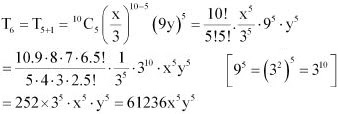Thus, the middle term in the expansion of (x/3 + 9y)10 is 61236 x5 y5.

9. In the expansion of (1 + a)m + n, prove that coefficients of am and an are equal.

Solution

It is known that (+ 1)th term, (Tr+1), in the binomial expansion of (b)n is given by Tr+1 = nCr an-r br .
Assuming that am occurs in the (r + 1)th term of the expansion (1 + a)m + n, we obtain
Tr+1 =  m+nCr (1)m+n-r (a)r . = m+nCr ar
Comparing the indices of a in am and in Tr+1, we obtain
r = m
Therefore, the coefficient of am is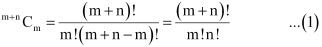Assuming that an occurs in the (k+1)th term of the expansion (1 + a)m+n, we obtain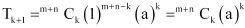Comparing the indices of a in an and in Tk+1, we obtain
k = n
Therefore, the coefficient of an is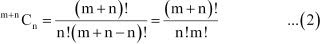Thus, from (1) and (2), it can be observed that the coefficients of am and an in the expansion of (1+ a)m+n are equal.

10. The coefficients of the (r – 1)thrth and (r + 1)th terms in the expansion of (x + 1)n are in the ratio 1:3:5. Find n and r.

Solution

It is known that (k +1)th term, (Tk+1 ) in the binomial expansion of (a + b)n is given by Tk+1 = nCk an-k bk .
Therefore, (r – 1)th term in the expansion of (x + 1)n is Tr-1 = nCr-2 (x)n-(r-2) (1)(r- 2) = nCr-2 Xn-r+2
rth term in the expansion of (x + 1)n is Tr = nCr-1 (x)n-(r-1) (1)(r-1) = nCr-1 xn-r+1
(r +1)th term in the expansion of (x +1)n is Tr+1 = nCr (X)n-r (1)r = nCr Xn-r
Therefore, the coefficients of the (r -1)th , rth and (r + 1)th terms in the expansion of (x + 1)n are nCr-2 , nCr-1 and nCr respectively. Since these coefficients are in the ratio 1 : 3 : 5, we obtain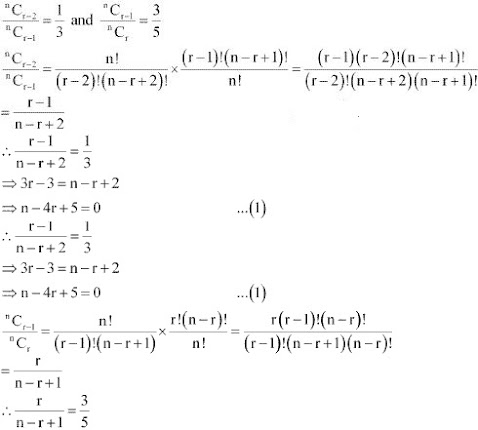Multiplying (1) by 3 and subtracting it from (2), we obtain
4– 12 = 0
⇒ r = 3
Putting the value of r in (1), we obtain
n – 12 + 5 = 0
⇒ n = 7
Thus, = 7 and r = 3

11. Prove that the coefficient of xn in the expansion of (1 + x)2n is twice the coefficient of xn in the expansion of (1 + x)2n–1 .

Solution

It is known that (+ 1)th term, (Tr+1), in the binomial expansion of (b)n is given by Tr+1 = nCr an-r br ..
Assuming that xn occurs in the (r + 1)th term of the expansion of (1 + x)2n, we obtain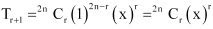Comparing the indices of x in xn and in Tr+1 , we obtain
r = n
Therefore, the coefficient of xn in the expansion of (1 + x)2n is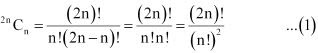Assuming that xn occurs in the (k +1)th term of the expansion (1 + x)2– 1, we obtain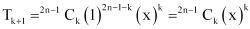Comparing the indices of x in xn and Tk + 1, we obtain
k = n
Therefore, the coefficient of xn in the expansion of (1 + x)2–1 is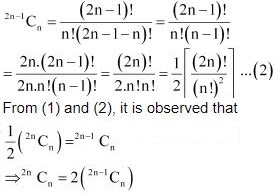Therefore, the coefficient of xn in the expansion of (1 + x)2n is twice the coefficient of xn in the expansion of (1 + x)2n–1.
Hence, proved.

12. Find a positive value of m for which the coefficient of x2 in the expansion (1 + x)m is 6

Solution

It is known that (+ 1)th term, (Tr+1), in the binomial expansion of (b)n is given by .
Tr+1 =  nCr an-r br .
Assuming that x2 occurs in the (+ 1)th term of the expansion (1 +x)m, we obtain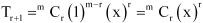Comparing the indices of x in x2 and in Tr + 1, we obtain
r = 2
Therefore, the coefficient of x2 is mC2 .
It is given that the coefficient of x2 in the expansion (1 + x)m is 6.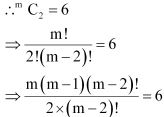⇒ m(m - 1) = 12
⇒ m2 - m - 12 = 0
⇒ m2 - 4m + 3m - 12 = 0
⇒ m(m - 4) + 3(m -4) = 0
⇒ (m - 4)(m + 3) = 0
⇒ (m - 4) = 0 or (m +3) = 0
⇒ m = 4 or m = -3
Thus, the positive value of m, for which the coefficient of x2 in the expansion
(1 + x)m is 6, is 4.